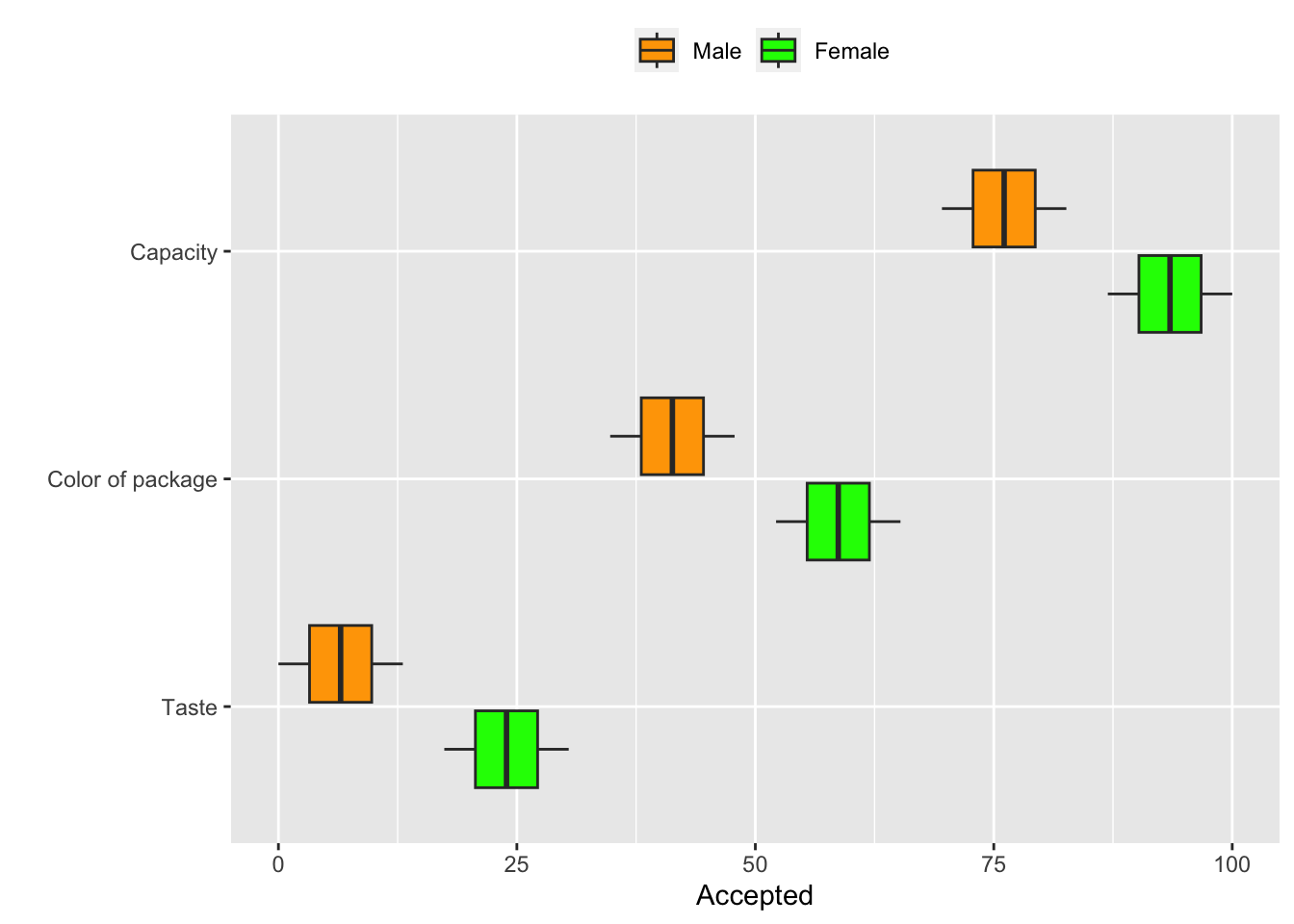# Customizing boxplots with ggplot2

Boxplot is probably one of the most common type of graphics. This will show how to customize boxplots.

You will learn,

• how to change the order of labels

• how to change the colours

• how to flip coordinates

Step 1: we create a simple data set.

Gender <- rep(c("Male", "Female"), times = 3, each = 4)
Quality <- rep(c("Taste", "Color of package", "Capacity"), times = 1, each = 8)
Accepted <- seq(0, 100, by = 100/23)

DF <- data.frame(Gender, Quality, Accepted)
head(DF)
##   Gender Quality  Accepted
## 1   Male   Taste  0.000000
## 2   Male   Taste  4.347826
## 3   Male   Taste  8.695652
## 4   Male   Taste 13.043478
## 5 Female   Taste 17.391304
## 6 Female   Taste 21.739130

Step 2: Generate a simple boxplot with ggplot

library(ggplot2)
ggplot(DF, aes(x = Quality, y = Accepted, fill = Gender)) +
geom_boxplot()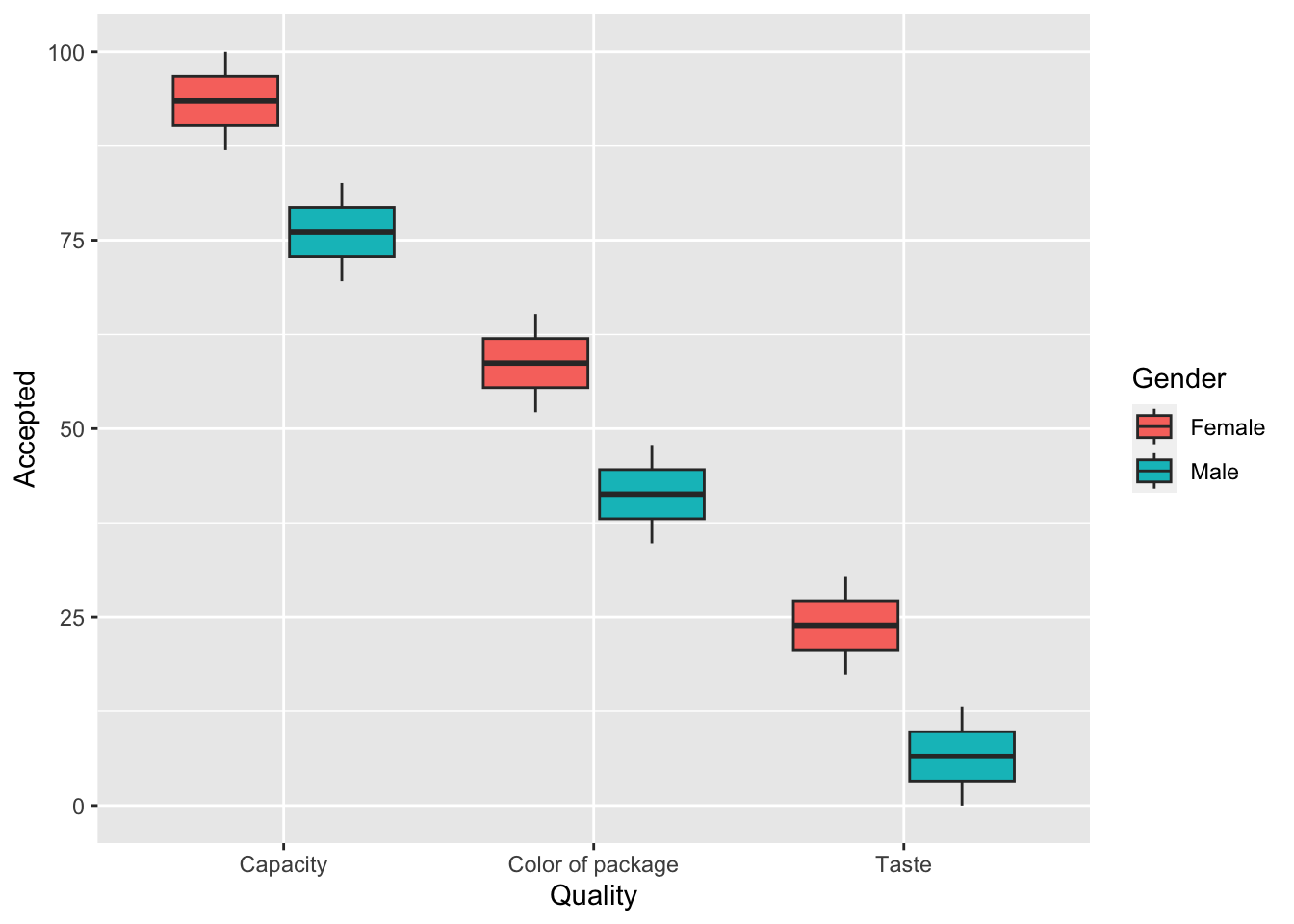Step 3: Change the colours, legend position and x-axis order

ggplot(DF, aes(x = Quality, y = Accepted, fill = Gender)) +
geom_boxplot() +
scale_fill_manual(values = c("green", "orange")) +
xlab("") +
theme(legend.position = "top", legend.title = element_blank()) +
scale_x_discrete(limits=c("Taste", "Color of package", "Capacity"))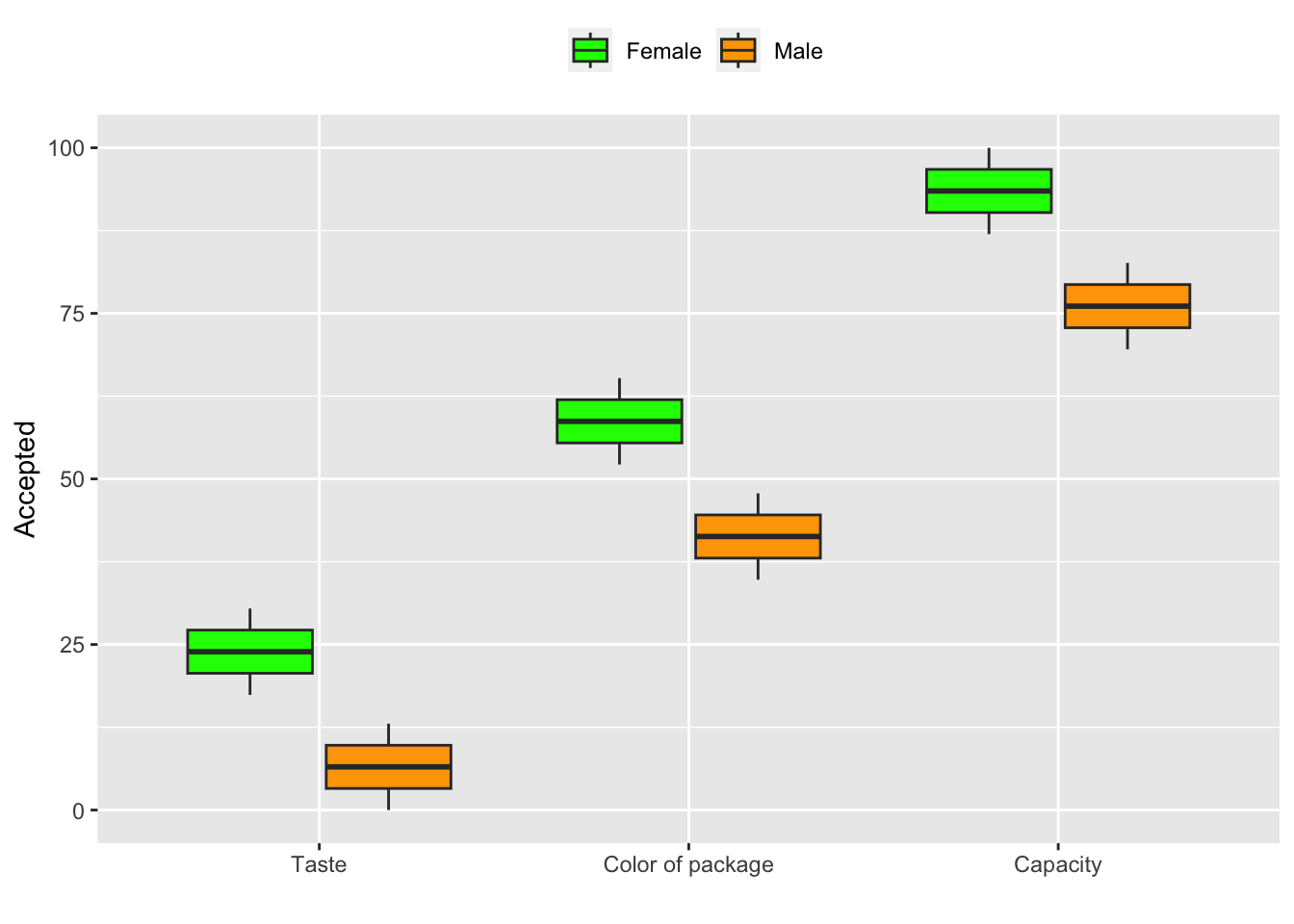Step 4: Change the plotting order: first you have to include factor code to set the order of the levels.

DF$Gender_factor <- factor(DF$Gender, levels=c("Male", "Female"))
ggplot(DF, aes(x = Quality, y = Accepted, fill = Gender_factor)) +
geom_boxplot() +
scale_fill_manual(values = c("green", "orange")) +
xlab("") +
theme(legend.position = "top", legend.title = element_blank()) +
scale_x_discrete(limits=c("Taste", "Color of package", "Capacity"))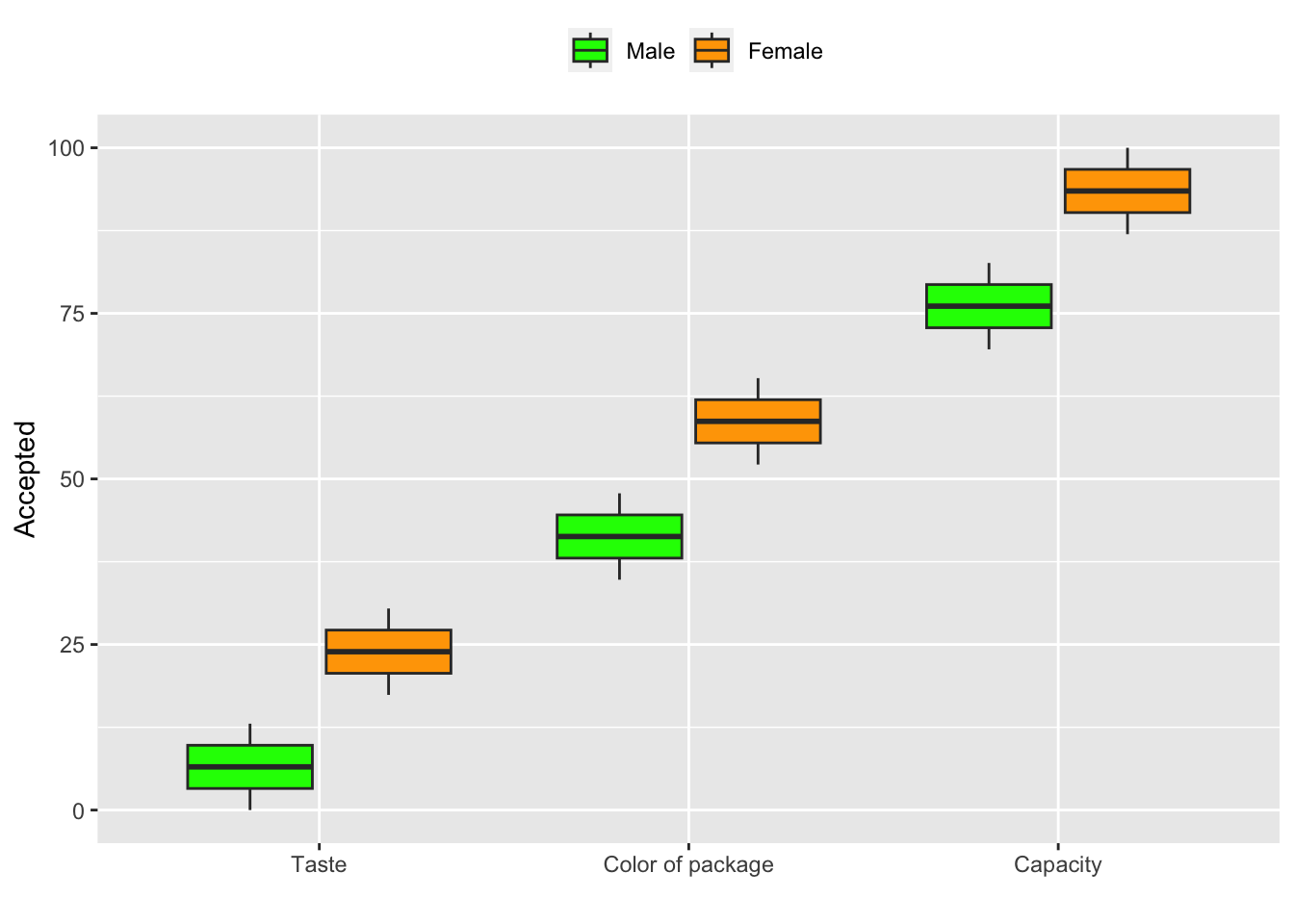Step 5: Horizontal bar chart: coord_flip()

ggplot(DF, aes(x = Quality, y = Accepted, fill = Gender)) +
geom_boxplot() +
scale_fill_manual(values = c("green", "orange")) +
xlab("") +
theme(legend.position = "top", legend.title = element_blank()) +
coord_flip()+
scale_x_discrete(limits=c("Taste", "Color of package", "Capacity"))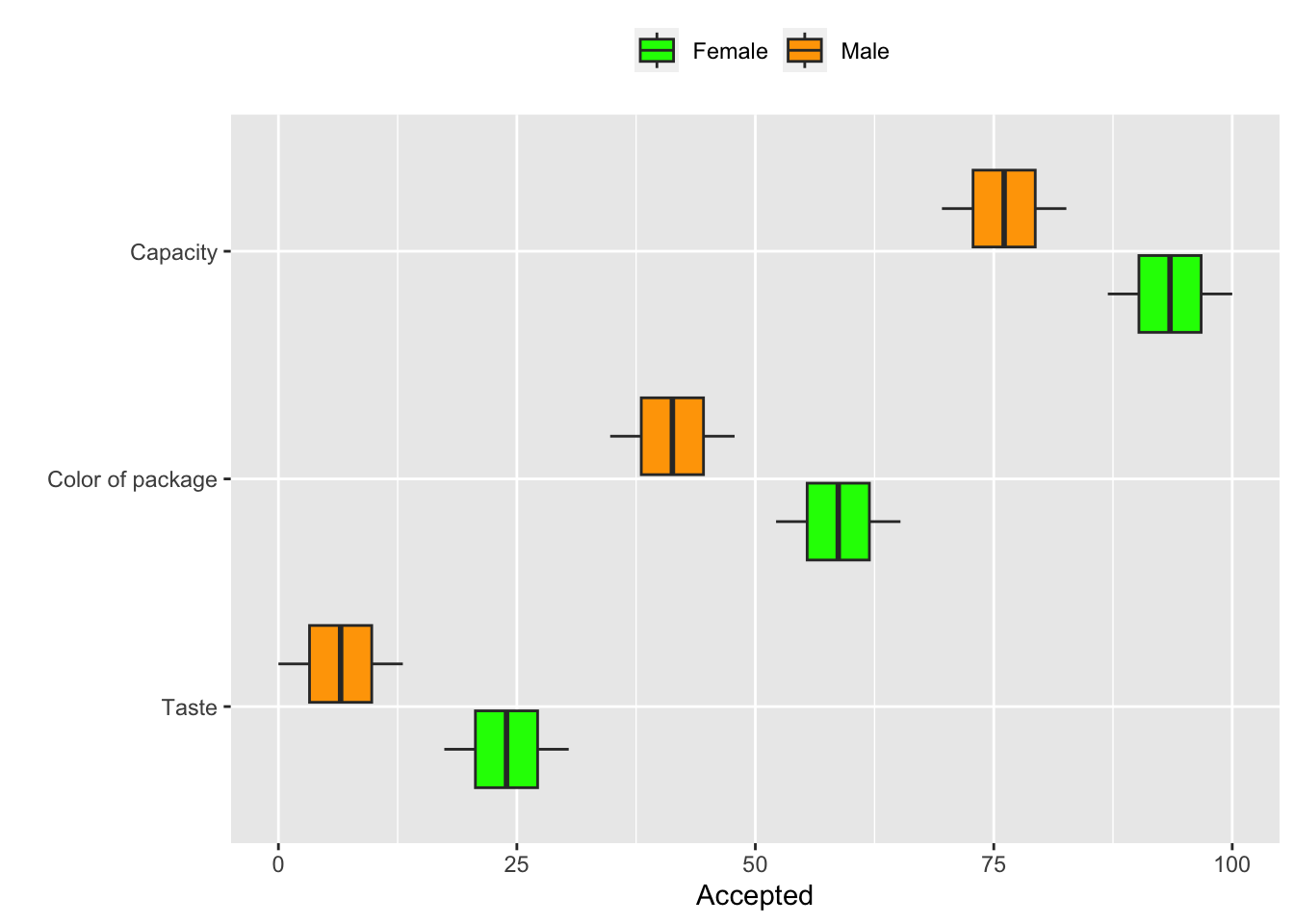step 6: Change the legend order similar to plot order: under themes fill=guide_legend(reverse=FALSE)

ggplot(DF, aes(x = Quality, y = Accepted, fill = Gender)) +
geom_boxplot() +
scale_fill_manual(values = c("green", "orange")) +
xlab("") +
theme(legend.position = "top", legend.title = element_blank()) +
guides(fill=guide_legend(reverse=TRUE)) +
coord_flip()+
scale_x_discrete(limits=c("Taste", "Color of package", "Capacity"))